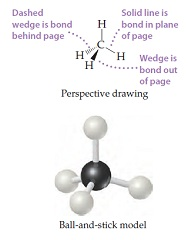# Problem: The methane molecule, CH4, has the geometry shown in following figure. Imagine a hypothetical process in which the methane molecule is "expanded," by simultaneously extending all four C–H bonds to infinity. We then have the process CH4(g) → C(g) + 4 H(g).Calculate the enthalpy change of formation of CH4(g)

###### FREE Expert Solution

We are asked to calculate the enthalpy change of formation of CH4(g)

Note that we need to multiply each ΔH˚ by the stoichiometric coefficient since ΔH˚ is in kJ/mol.

Balanced reaction : C(s) + 2 H2(g) CH4(g).

91% (293 ratings)###### Problem Details
The methane molecule, CH4, has the geometry shown in following figure. Imagine a hypothetical process in which the methane molecule is "expanded," by simultaneously extending all four C–H bonds to infinity. We then have the process CH4(g) → C(g) + 4 H(g).Calculate the enthalpy change of formation of CH4(g)

Frequently Asked Questions

What scientific concept do you need to know in order to solve this problem?

Our tutors have indicated that to solve this problem you will need to apply the Enthalpy of Formation concept. You can view video lessons to learn Enthalpy of Formation. Or if you need more Enthalpy of Formation practice, you can also practice Enthalpy of Formation practice problems.